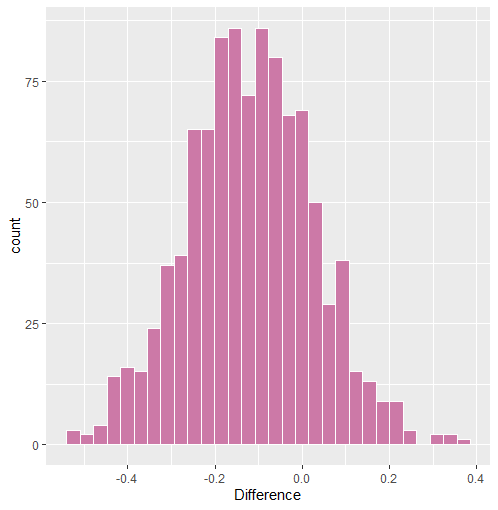# Introduction

This is a follow-up to the vignette “Three Ways to Test the Same Hypothesis”. A new feature, pcor_sum, was added to BGGM that allows for testing partial correlation sums. This differs from the Bayes factor approach (“Approach #3”), in that only the posterior distribution is used to determine whether there is a difference in the sums.

### R package

# need the developmental version
if (!requireNamespace("remotes")) {
install.packages("remotes")
}

# install from github
remotes::install_github("donaldRwilliams/BGGM")
library(BGGM)

# One Group

This first example looks at one group, where a sum is tested within the same ptsd network. I focus on the relations between the re-experiencing (B) and avoidance (C) communities. In particular, the sum of relations between the “Intrusion” (5 nodes) community and the “Avoidance” (two nodes) community is tested.

## Sum to String

For the avoidance symptom “avoidance of thoughts” C1, this can be written in R code with

# ptsd
Y <- ptsd

# paste together sums
paste0(colnames(Y)[1:5],  "--C1", collapse = " + ")

#> "B1--C1 + B2--C1 + B3--C1 + B4--C1 + B5--C1"

whereas, for the avoidance symptom “avoidance of reminders” (C2), this is written as

paste0(colnames(Y)[1:5],  "--C2", collapse = " + ")

#> "B1--C2 + B2--C2 + B3--C2 + B4--C2 + B5--C2"

Note that typically this would have to be written out. paste0 was used in this case to avoid typing out all of the relations.

## Fit Model

Here an ordinal GGM is fitted

fit <- estimate(Y+1, type = "ordinal", iter = 1000)

where the +1 changes the first category from 0 to 1 (required).

## Test Sums

The next step is to use the pcor_sum function. First, I combine the sums into one string separated with ;.

# sum 1
sum1 <- paste0(colnames(Y)[1:5],  "--C1", collapse = " + ")

# sum 2
sum2 <- paste0(colnames(Y)[1:5],  "--C2", collapse = " + ")

# paste together
sums <- paste(sum1, sum2, sep = ";")

# print
sums
#> "B1--C1 + B2--C1 + B3--C1 + B4--C1 + B5--C1;B1--C2 + B2--C2 + B3--C2 + B4--C2 + B5--C2"

Next pcor_sum is used

test_sum <- pcor_sum(fit, relations = sums)

# print
test_sum

# BGGM: Bayesian Gaussian Graphical Models
# ---
# Network Stats: Posterior Sum
# Posterior Samples: 1000
# ---
# Estimates
#
# Sum:
#                                    Post.mean Post.sd Cred.lb Cred.ub
# B1--C1+B2--C1+B3--C1+B4--C1+B5--C1     0.215   0.096   0.034   0.404
# B1--C2+B2--C2+B3--C2+B4--C2+B5--C2     0.334   0.097   0.145   0.514
# ---
#
# Difference:
# B1--C1+B2--C1+B3--C1+B4--C1+B5--C1 - B1--C2+B2--C2+B3--C2+B4--C2+B5--C2
#
#  Post.mean Post.sd Cred.lb Cred.ub Prob.greater Prob.less
#     -0.119   0.145  -0.409   0.173        0.205     0.795
# --- 

Prob.greater is the posterior probability that the first sum is larger than the second sum.

## Plot Results

The object test_sum can then be plotted. Note this returns three plots, but only the difference is shown here

plot(test_sum)\$diffThe histogram is not very smooth in this case because iter = 1000, but this of course can be changed.

# Two Groups

This next example is for two groups. The data are called bfi and they are in the BGGM package. I compare a sum of two relations for questions measuring agreeableness in males and females. The relations tested are as follows

## Sum to String

sums <- c("A3--A4 + A4--A5")

where A1 is “know how to comfort others”, A4 is “love children”, and A5 is “make people feel at ease”.

## Fit Models

The next step is to fit the models

# data
Y <- bfi

# males
Y_males <- subset(Y, gender == 1, select = -c(education, gender))[,1:5]

# females
Y_females <- subset(Y, gender == 2, select = -c(education, gender))[,1:5]

fit_female <- estimate(Y_females, seed = 2)

# fit males
fit_male <- estimate(Y_males, seed = 1)

## Test Sums

Then test the sum

test_sum <- pcor_sum(fit_female, fit_male, relations = sums)

# print
test_sum

#> BGGM: Bayesian Gaussian Graphical Models
#> ---
#> Network Stats: Posterior Sum
#> Posterior Samples: 5000
#> ---
#> Estimates
#>
#> Sum:
#>                   Post.mean Post.sd Cred.lb Cred.ub
#> g1: A3--A4+A4--A5     0.292   0.026   0.241   0.342
#> g2: A3--A4+A4--A5     0.305   0.036   0.234   0.375
#> ---
#>
#> Difference:
#> g1: A3--A4+A4--A5 - g2: A3--A4+A4--A5
#>
#>  Post.mean Post.sd Cred.lb Cred.ub Prob.greater Prob.less
#>     -0.014   0.045    -0.1   0.074        0.386     0.614
#> --- 

## Sanity Check

For a kind of sanity check, here is the sum for the male group obtained from the point estimates.

pcor_mat(fit_male)["A3", "A4"] + pcor_mat(fit_male)["A4", "A5"]

#>  0.305

This matches the output.